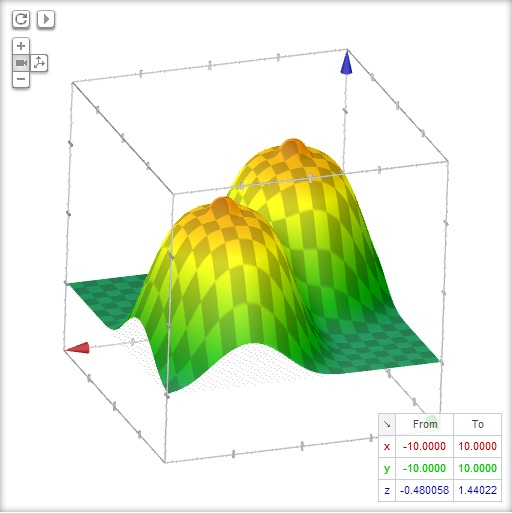## Browse By

So, I came across a rather interesting equation. The equation can be used to plot a rather "interesting" 3D graph. You don't need any hi-tech scientific tools to plot this. Just go over google.com and copy paste this equation:

exp(-((x-4)^2+(y-4)^2)^2/1000) + exp(-((x+4)^2+(y+4)^2)^2/1000) + 0.1exp(-((x+4)^2+(y+4)^2)^2)+0.1exp(-((x -4)^2+(y-4)^2)^2)

Come back and tell me what you think 😛#### Incoming search terms:

If you liked this article, feel free to share it. It will help us a lot!
• 1
•
•
•
•
•
•
•
•
1
Share
• 1
•
•
•
•
•
•
•
•

This site uses Akismet to reduce spam. Learn how your comment data is processed.